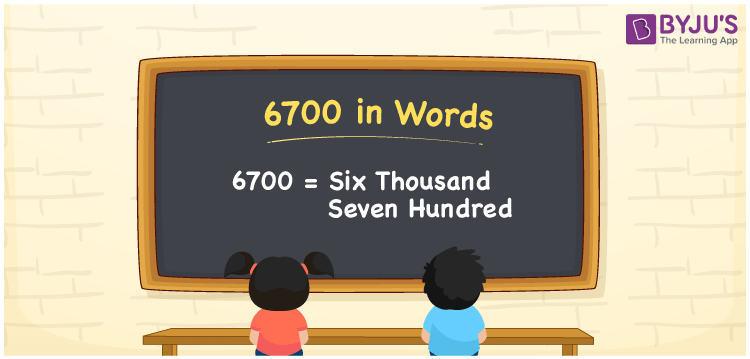# 6700 in Words

6700 in words can be written as Six Thousand Seven Hundred. For instance, Meera purchased a saree worth Rs. 6700, then you can say, “Meera purchased a saree worth Rupees Six Thousand Seven Hundred”. The number 6700 is a cardinal number as it represents a specific quantity. In this article, we will learn the conversion of the number 6700 into words using the English alphabet.

 6700 in Words Six Thousand Seven Hundred Six Thousand Seven Hundred in numerical form 6700

## 6700 in English Words

Generally, in Maths, students write the numbers in words using the English alphabet. Therefore, we can read the number 6700 in English as Six Thousand Seven Hundred.## How to Write 6700 in Words?

Find the position of each digit in the number 6700 and then convert it into words. The below table shows the place value chart for the number 6700 with 4 columns since 6700 is a four-digit number.

 Thousands Hundreds Tens Ones 6 7 0 0

Hence, we can write the expanded form as:

6 x Thousand + 7 x Hundred + 0 x Ten + 0 x One

= 6 x 1000 + 7 x 100 + 0 x 10 + 0 x 1

= 6000 + 700 + 0 + 0

= 6000 + 700

= 6700

= Six Thousand Seven Hundred

Therefore, 6700 in words is written as Six Thousand Seven Hundred

Interesting way of writing 6700 in words

6 = Six

67 = Sixty-Seven

670 = Six Hundred and Seventy

6700 = Six Thousand Seven Hundred

Thus, the word form of the number 6700 is Six Thousand Seven Hundred

6700 is a natural number that precedes 6701 and succeeds 6699

• 6700 in words – Six Thousand Seven Hundred
• Is 6700 an odd number? – No
• Is 6700 an even number? – Yes
• Is 6700 a perfect square number? – No
• Is 6700 a perfect cube number? – No
• Is 6700 a prime number? – No
• Is 6700 a composite number? – Yes

## Frequently Asked Questions on 6700 in Words

Q1

### How to write 6700 in words?

We can write 6700 in words as Six Thousand Seven Hundred.
Q2

### Simplify 5000 + 1700, and express in words.

Simplifying 5000 + 1700, we get 6700. Hence, 6700 in words is Six Thousand Seven Hundred.
Q3

### Is 6700 an even number?

Yes, the number 6700 is an even number.